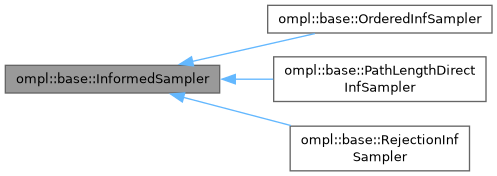ompl::base::InformedSampler Class Referenceabstract

An abstract class for the concept of using information about the state space and the current solution cost to limit future search to a planning subproblem that contains all possibly better solutions. More...

#include <ompl/base/samplers/InformedStateSampler.h>

Inheritance diagram for ompl::base::InformedSampler:[legend]

## Public Member Functions

InformedSampler (const InformedSampler &)=delete

InformedSampleroperator= (const InformedSampler &)=delete

InformedSampler (const ProblemDefinitionPtr &probDefn, unsigned int maxNumberCalls)
Construct a sampler that only generates states with a heuristic solution estimate that is less than the cost of the current solution. Requires a function pointer to a method to query the cost of the current solution. If iteration is required, only maxNumberCalls are attempted, to assure that the function returns.

virtual bool sampleUniform (State *statePtr, const Cost &maxCost)=0
Sample uniformly in the subset of the state space whose heuristic solution estimates are less than the provided cost, i.e. in the interval [0, maxCost). Returns false if such a state was not found in the specified number of iterations.

virtual bool sampleUniform (State *statePtr, const Cost &minCost, const Cost &maxCost)=0
Sample uniformly in the subset of the state space whose heuristic solution estimates are between the provided costs, [minCost, maxCost). Returns false if such a state was not found in the specified number of iterations.

virtual bool hasInformedMeasure () const =0
Whether the sampler can provide a measure of the informed subset.

virtual double getInformedMeasure (const Cost &currentCost) const =0
The measure of the subset of the state space defined by the current solution cost that is being searched. Does not consider problem boundaries but returns the measure of the entire space if no solution has been found or if a closed form expression for the measure does not exist.

virtual double getInformedMeasure (const Cost &minCost, const Cost &maxCost) const
The measure of the subset of the state space defined by the current solution cost that is being searched. Does not consider problem boundaries but returns the measure of the entire space if no solution has been found or if a closed form expression for the measure does not exist. By default calls the 1-argument overloaded version with the min and max costs and subtracts the differences; however, there may be more efficient ways to do this for some cost functions.

virtual Cost heuristicSolnCost (const State *statePtr) const
A helper function to calculate the heuristic estimate of the solution cost for a given state using the optimization objective stored in the problem definition. More...

ProblemDefinitionPtr getProblemDefn () const

unsigned int getMaxNumberOfIters () const

## Protected Attributes

ProblemDefinitionPtr probDefn_
A copy of the problem definition.

OptimizationObjectivePtr opt_
A copy of the optimization objective.

StateSpacePtr space_
A copy of the state space.

unsigned int numIters_
The number of iterations I'm allowed to attempt.

## Detailed Description

An abstract class for the concept of using information about the state space and the current solution cost to limit future search to a planning subproblem that contains all possibly better solutions.

Definition at line 60 of file InformedStateSampler.h.

## ◆ getMaxNumberOfIters()

 unsigned int ompl::base::InformedSampler::getMaxNumberOfIters ( ) const

Helper for the OrderedInfSampler wrapper

Definition at line 115 of file InformedStateSampler.cpp.

## ◆ getProblemDefn()

 ProblemDefinitionPtr ompl::base::InformedSampler::getProblemDefn ( ) const

Helper for the OrderedInfSampler wrapper

Definition at line 110 of file InformedStateSampler.cpp.

## ◆ heuristicSolnCost()

 Cost ompl::base::InformedSampler::heuristicSolnCost ( const State * statePtr ) const
virtual

A helper function to calculate the heuristic estimate of the solution cost for a given state using the optimization objective stored in the problem definition.

Todo:
With the future invention of a heuristic class, this should move.

Reimplemented in ompl::base::PathLengthDirectInfSampler.

Definition at line 77 of file InformedStateSampler.cpp.

The documentation for this class was generated from the following files: